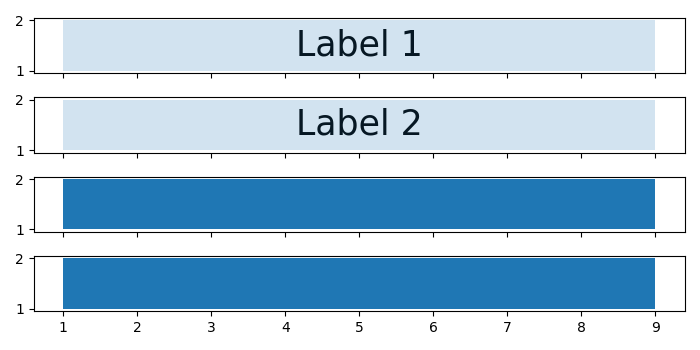# How to better rasterize a plot without blurring the labels in matplotlib?

To rasterize a plot in a bettery way without blurring the labels in matplotlib, we can take the following steps.

## Steps

• Set the figure size and adjust the padding between and around the subplots.

• Create a figure and a set of subplots.

• Axis 0 – Fill the area between the curve with alpha and rasterized=False.

• Add text to the axes.

• Axis 1 – Fill the area between the curve with alpha and rasterized=True.

• Add text to the axes.

• Axes 2 and 3 – Fill the area between the curve without alpha and rasterized=True and False, respectively.

• Add text to the axes.

• To display the figure, use Show() method.

## Example

import matplotlib.pyplot as plt
import numpy as np

plt.rcParams["figure.figsize"] = [7.00, 3.50]
plt.rcParams["figure.autolayout"] = True

fig, axes = plt.subplots(nrows=4, sharex=True)

axes.fill_between(np.arange(1, 10), 1, 2, zorder=-1, alpha=0.2, rasterized=False)
axes.text(5, 1.5, "Label 1", ha='center', va='center', fontsize=25, zorder=-2, rasterized=True)

axes.fill_between(np.arange(1, 10), 1, 2, zorder=-1, alpha=0.2, rasterized=True)
axes.text(5, 1.5, "Label 2", ha='center', va='center', fontsize=25, zorder=-2, rasterized=True)

axes.fill_between(np.arange(1, 10), 1, 2, zorder=-1, rasterized=True)
axes.text(5, 1.5, "Label 3", ha='center', va='center', fontsize=25, zorder=-2, rasterized=True)

axes.fill_between(np.arange(1, 10), 1, 2, zorder=-1, rasterized=False)
axes.text(5, 1.5, "Label 4", ha='center', va='center', fontsize=25, zorder=-2, rasterized=True)

plt.show()

## Output

It will produce the following output −Observe that, since we have not used any "alpha" on axes 2 and 3, the labels are not visible.